# Sharing \$15.75 Worth of Puzzles

### Pattern Puzzle:

When I saw that 35 × 45 = 1575, I suspected a pattern. I made a chart to see if my suspicions were true, and they were! Can you look at the chart and tell me what that pattern is?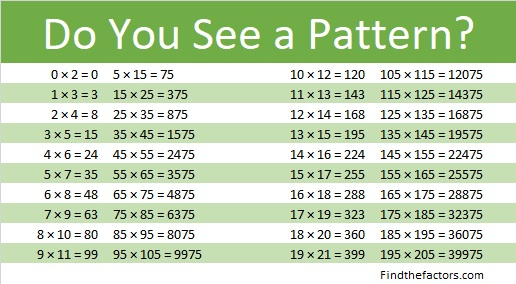If you were able to see that pattern, then look at each of these. They have patterns because the numbers in 3 × 17, 4 × 16, 5 × 15, 6 × 14, and 7 × 13 have a relationship. What is that relationship?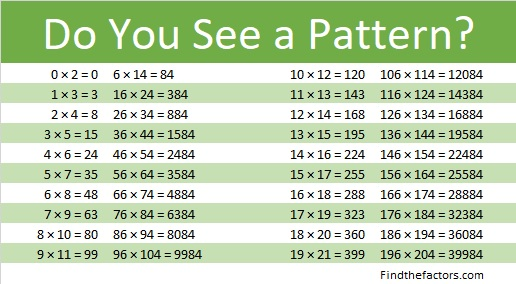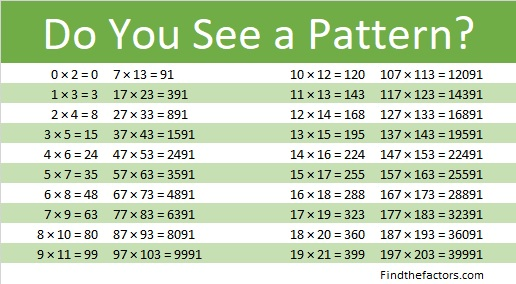### Factors of 1575:

We can use 35 × 45 = 1575 to make one of its many possible factor trees:• 1575 is a composite number.
• Prime factorization: 1575 = 3 × 3 × 5 × 5 × 7, which can be written 1575 = 3² × 5² × 7.
• 1575 has at least one exponent greater than 1 in its prime factorization so √1575 can be simplified. Taking the factor pair from the factor pair table below with the largest square number factor, we get √1575 = (√225)(√7) = 15√7.
• The exponents in the prime factorization are 2, 2, and 1. Adding one to each exponent and multiplying we get (2 + 1)(2 + 1)(1 + 1) = 3 × 3 × 2 = 18. Therefore 1575 has exactly 18 factors.
• The factors of 1575 are outlined with their factor pair partners in the graphic below.Can you use a different factor pair to create another factor tree for 1575? Will you always get 1575 = 3² × 5² × 7 in the end?

### Difference of Two Squares Puzzle:

1575 is the difference of two squares in NINE different ways:
788² – 787² = 1575,
264² – 261² = 1575,
160² – 155² = 1575,
116² – 109² = 1575,
92² – 83² = 1575,
60² – 45² = 1575,
48² – 27² = 1575,
44² – 19² = 1575, and
40² – 5² = 1575.

In money 1575¢ is represented as \$15.75. That’s the same as 63 quarters! Which of those differences of two squares is illustrated using quarters in the image below:Which of the nine difference of two squares above is illustrated in the following image?That image illustrates that \$15.75 is just one quarter away from the next perfect square dollar amount, \$16.00. Both 16 and 1600 are perfect squares. Can you make the rectangle below by moving just one row of quarters from the image above?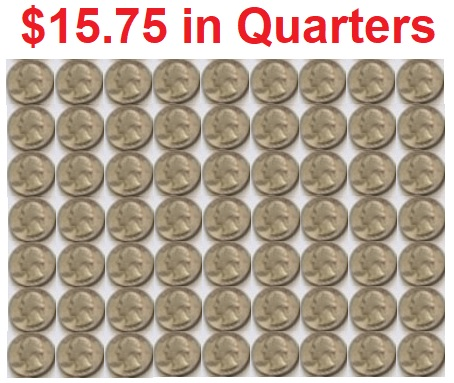8² – 1² = (8 – 1) × (8 + 1) = 63,
and might be the first step in understanding that  a² – b² = (a + b)(a – b) .

### Dividing Mixed Numbers Puzzles:

A quarter is 25¢. The reason a quarter is called a quarter is because it is a quarter or 1/4th of a dollar. We usually write dollar and cents together as decimals. A quarter is \$0.25.

Three quarters is 75¢ or \$0.75 and is 3/4ths of a dollar.

Two quarters is 50¢ or \$0.50 and is 2/4ths or one half of a dollar.

Representing 1575¢ in quarters can help you understand dividing mixed numbers like in the problem below: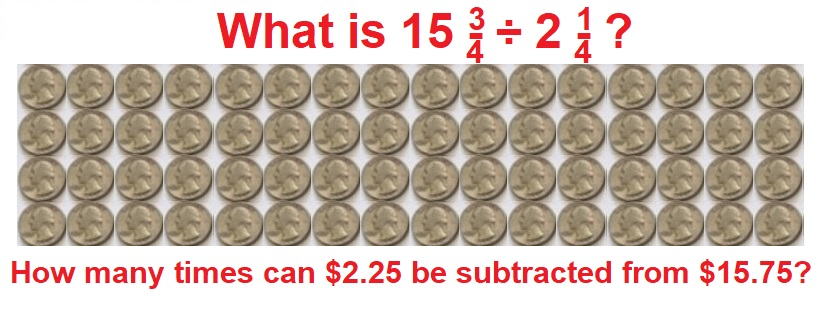You might not find this next example easy, but give it a look:Why is 13 in the denominator of the answer to both questions when it didn’t appear in either question? Where did the 13 come from?

Now try writing and solving your own problem:Working with money often seems like more fun than working with numbers. I hope you enjoyed these puzzles today.